Courses

# Pipes and Cisterns - Examples (with Solutions), Logical Reasoning CAT Notes | EduRev

## CAT : Pipes and Cisterns - Examples (with Solutions), Logical Reasoning CAT Notes | EduRev

The document Pipes and Cisterns - Examples (with Solutions), Logical Reasoning CAT Notes | EduRev is a part of CAT category.
All you need of CAT at this link: CAT

1. Pipes A and B can fill a tank in 5 and 6 hours respectively. Pipe C can empty it in 12 hours. If all the three pipes are opened together, then the tank will be filled in: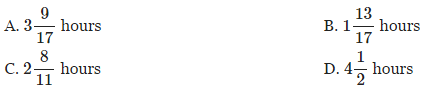Explanation:

Solution 1

Pipes A and B can fill the tank in 5 and 6 hours respectively. Therefore,
part filled by pipe A in 1 hour =1/5
part filled by pipe B in 1 hour = 1/6

Pipe C can empty the tank in 12 hours. Therefore,
part emptied by pipe C in 1 hour =1/12

Net part filled by Pipes A,B,C together in 1 hour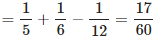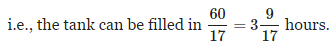Solution 2

LCM(5,6,12)=60

Suppose capacity of the tank is 60 litre. Then,
Quantity filled by pipe A in 1 hour = 60/5=12 litre.
Quantity filled by pipe B in 1 hour = 60/6=10 litre.
Quantity emptied by pipe C in 1 hour = 60/12=5 litre.

Quantity filled in 1 hour if all the pipes are opened together
=12+10-5=17 litre.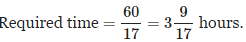2. Two pipes A and B can fill a cistern in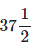minutes and 45 minutes respectively. Both pipes are opened. The cistern will be filled in just half an hour, if pipe B is turned off after

A. 5 min

B. 9 min

C. 10 min

D. 15 min

Explanation:

Pipe A alone can fill the cistern in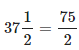minutes. Since it was open for 3030 minutes, part of the cistern filled by pipe A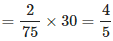So the remaining 1/5 part is filled by pipe B.

Pipe B can fill the cistern in 45 minutes. So, time required to fill 1/5 part
=45/5=9 minutes.

i.e., pipe B is turned off after 9 minutes.

3. A pump can fill a tank with water in 2 hours. Because of a leak, it took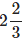hours to fill the tank. The leak can drain all the water of the tank in

A. 6 hours

B. 8 hours

C. 9 hours

D. 10 hours

Explanation:

The pump can fill the tank in 2 hour. But, because of the leak, it tookhours to fill the tank.

i.e., the additional water filled by the pump in 2/3 hour is emptied by the leak in=8/3 hours.

Therefore, the water filled by pump in 2 bour can be emptied by the leak in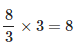= 8 hours.

i.e., The leak can drain all the water of the tank in 8 hours.

4. Three pipes A, B and C can fill a tank from empty to full in 30 minutes, 20 minutes, and 10 minutes respectively. When the tank is empty, all the three pipes are opened. A, B and C discharge chemical solutions P,Q and R respectively. What is the proportion of the solution R in the liquid in the tank after 3 minutes?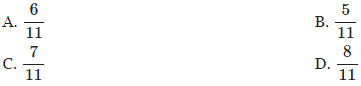Explanation:

Part of the tank filled by pipe A in 1 minute =1/30
Part of the tank filled by pipe B in 1 minute =1/20
Part of the tank filled by pipe C in 1 minute =1/10

Here we have to find the proportion of the solution R.

Pipe C discharges chemical solution R.

Part of the tank filled by pipe C in 3 minutes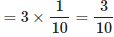Part of the tank filled by pipe A,B,C together in 1 minutePart of the tank filled by pipe A,B,C together in 3 minute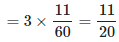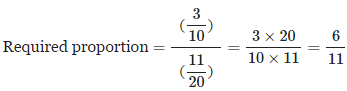5. A tank is filled by three pipes with uniform flow. The first two pipes operating simultaneously fill the tank in the same time during which the tank is filled by the third pipe alone. The second pipe fills the tank 5 hours faster than the first pipe and 4 hours slower than the third pipe. Time required by the first pipe to fill the tank is

A. 30 hours

B. 15 hours

C. 10 hours

D. 6 hours

Explanation:

Suppose the first pipe alone can fill the tank in x hours. Then,
second pipe alone can fill the tank in (x-5) hours,
third pipe alone can fill the tank in (x-5) -4=(x-9) hours.

Part filled by first pipe and second pipe together in 1 hr
= Part filled by third pipe in 1 hr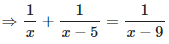From here, better to find the value of xx from the given choices which will be easier. Or we can solve it as follows.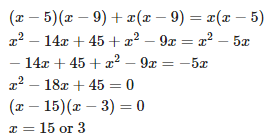We can not take the value x=3because, (x-9) becomes negative which is not possible, because the third pipe can fill the tank in (x-9) hours.

Hence, x=15

Offer running on EduRev: Apply code STAYHOME200 to get INR 200 off on our premium plan EduRev Infinity!

,

,

,

,

,

,

,

,

,

,

,

,

,

,

,

,

,

,

,

,

,

,

,

,

;